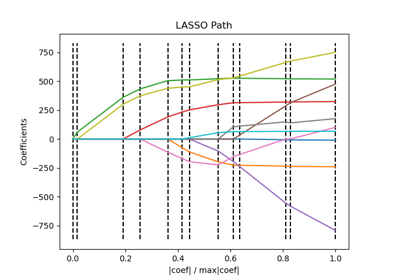# sklearn.linear_model.lars_path¶

sklearn.linear_model.lars_path(X, y, Xy=None, *, Gram=None, max_iter=500, alpha_min=0, method='lar', copy_X=True, eps=2.220446049250313e-16, copy_Gram=True, verbose=0, return_path=True, return_n_iter=False, positive=False)[source]

Compute Least Angle Regression or Lasso path using LARS algorithm 

The optimization objective for the case method=’lasso’ is:

(1 / (2 * n_samples)) * ||y - Xw||^2_2 + alpha * ||w||_1


in the case of method=’lars’, the objective function is only known in the form of an implicit equation (see discussion in )

Read more in the User Guide.

Parameters
XNone or array-like of shape (n_samples, n_features)

Input data. Note that if X is None then the Gram matrix must be specified, i.e., cannot be None or False.

yNone or array-like of shape (n_samples,)

Input targets.

Xyarray-like of shape (n_samples,) or (n_samples, n_targets), default=None

Xy = np.dot(X.T, y) that can be precomputed. It is useful only when the Gram matrix is precomputed.

GramNone, ‘auto’, array-like of shape (n_features, n_features), default=None

Precomputed Gram matrix (X’ * X), if 'auto', the Gram matrix is precomputed from the given X, if there are more samples than features.

max_iterint, default=500

Maximum number of iterations to perform, set to infinity for no limit.

alpha_minfloat, default=0

Minimum correlation along the path. It corresponds to the regularization parameter alpha parameter in the Lasso.

method{‘lar’, ‘lasso’}, default=’lar’

Specifies the returned model. Select 'lar' for Least Angle Regression, 'lasso' for the Lasso.

copy_Xbool, default=True

If False, X is overwritten.

epsfloat, optional

The machine-precision regularization in the computation of the Cholesky diagonal factors. Increase this for very ill-conditioned systems. By default, np.finfo(np.float).eps is used.

copy_Grambool, default=True

If False, Gram is overwritten.

verboseint, default=0

Controls output verbosity.

return_pathbool, default=True

If return_path==True returns the entire path, else returns only the last point of the path.

return_n_iterbool, default=False

Whether to return the number of iterations.

positivebool, default=False

Restrict coefficients to be >= 0. This option is only allowed with method ‘lasso’. Note that the model coefficients will not converge to the ordinary-least-squares solution for small values of alpha. Only coefficients up to the smallest alpha value (alphas_[alphas_ > 0.].min() when fit_path=True) reached by the stepwise Lars-Lasso algorithm are typically in congruence with the solution of the coordinate descent lasso_path function.

Returns
alphasarray-like of shape (n_alphas + 1,)

Maximum of covariances (in absolute value) at each iteration. n_alphas is either max_iter, n_features or the number of nodes in the path with alpha >= alpha_min, whichever is smaller.

activearray-like of shape (n_alphas,)

Indices of active variables at the end of the path.

coefsarray-like of shape (n_features, n_alphas + 1)

Coefficients along the path

n_iterint

Number of iterations run. Returned only if return_n_iter is set to True.

References

1

“Least Angle Regression”, Efron et al. http://statweb.stanford.edu/~tibs/ftp/lars.pdf

2

Wikipedia entry on the Least-angle regression

3

Wikipedia entry on the Lasso

## Examples using sklearn.linear_model.lars_path¶